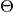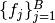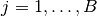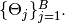# Model¶

The `Model` type is designed to store the set of all model nodes, including parameter setas denoted in the Mamba Gibbs sampling scheme. In particular, it stores `Dependent` type objects in its `nodes` dictionary field. Valid models are ones whose nodes form directed acyclic graphs (DAGs). Sampling functionsare saved as `Sampler` objects in the vector of field `samplers`. Vector elementscorrespond to sampling blocks## Declaration¶

`type Model`

## Fields¶

• `nodes::Dict{Symbol,Any}` : a dictionary containing all input, logical, and stochastic model nodes.
• `dependents::Vector{Symbol}` : symbols of all `Dependent` nodes in topologically sorted order so that a given node in the vector is conditionally independent of subsequent nodes, given the previous ones.
• `samplers::Vector{Sampler}` : sampling functions for updating blocks of stochastic nodes.
• `states::Vector{Vector{VariateType}}` : states of chains at the end of a possible series of MCMC runs.
• `iter::Integer` : current MCMC draw from the target distribution.
• `burnin::Integer` : number of initial draws to discard as a burn-in sequence to allow for convergence.
• `chain::Integer` : current run of an MCMC simulator in a possible series of runs.
• `hasinputs::Bool` : whether values have been assigned to the input nodes.
• `hasinits::Bool` : whether initial values have been assigned to stochastic nodes.

## Constructor¶

`Model`(; iter::Integer=0, burnin::Integer=0, chain::Integer=1, samplers::Vector{Sampler}=Array(Sampler, 0), nodes...)

Construct a `Model` object that defines a model for MCMC simulation.

Arguments

• `iter` : current iteration of the MCMC simulation.
• `burnin` : number of initial draws to be discarded as a burn-in sequence to allow for convergence.
• `chain` : current run of the MCMC simulator in a possible sequence of runs.
• `samplers` : a vector of block-specific sampling functions.
• `nodes...` : an arbitrary number of user-specified arguments defining logical and stochastic nodes in the model. Argument values must be `Logical` or `Stochastic` type objects. Their names in the model will be taken from the argument names.

Value

Returns a `Model` type object.

Example

See the Model Specification section of the tutorial.

## Methods¶

`draw`(m::Model; filename::String="")

Draw a GraphViz DOT-formatted graph representation of model nodes and their relationships.

Arguments

• `m` : a model for which to construct a graph.
• `filename` : an external file to which to save the resulting graph, or an empty string to draw to standard output (default). If a supplied external file name does not include a dot (`.`), the file extension `.dot` will be appended automatically.

Value

The model drawn to an external file or standard output. Stochastic, logical, and input nodes will be represented by ellipses, diamonds, and rectangles, respectively. Nodes that are unmonitored in MCMC simulations will be gray-colored.

Example

See the Directed Acyclic Graphs section of the tutorial.
`getindex`(m::Model, key::Symbol)

Returns a model node identified by its symbol. The syntax `m[key]` is converted to `getindex(m, key)`.

Arguments

• `m` : a model contining the node to get.
• `key` : symbol of the node to get.

Value

The specified node.
`gradlogpdf`(m::Model, block::Integer=0, transform::Bool=false; dtype::Symbol=:forward)
`gradlogpdf`(m::Model, x::Vector{T<:Real}, block::Integer=0, transform::Bool=false; dtype::Symbol=:forward)
`gradlogpdf!`(m::Model, x::Vector{T<:Real}, block::Integer=0, transform::Bool=false; dtype::Symbol=:forward)

Compute the gradient of log-densities for stochastic nodes.

Arguments

• `m` : a model containing the stochastic nodes for which to compute the gradient.

• `x` : a value (possibly different than the current one) at which to compute the gradient.

• `block` : the sampling block of stochastic nodes for which to compute the gradient, if specified; otherwise, all sampling blocks are included.

• `transform` : whether to compute the gradient of block parameters on the link–transformed scale.

• `dtype` : type of differentiation for gradient calculations. Options are
• `:central` : central differencing.
• `:forward` : forward differencing.

Value

The resulting gradient vector. Method `gradlogpdf!()` additionally updates model `m` with supplied values `x`.

Note

Numerical approximation of derivatives by central and forward differencing is performed with the Calculus package .
`graph`(m::Model)

Construct a graph representation of model nodes and their relationships.

Arguments

• `m` : a model for which to construct a graph.

Value

Returns a `GenericGraph` type object as defined in the Graphs package.
`graph2dot`(m::Model)

Draw a GraphViz DOT-formatted graph representation of model nodes and their relationships.

Arguments

• `m` : a model for which to construct a graph.

Value

A character string representation of the graph suitable for in-line processing. Stochastic, logical, and input nodes will be represented by ellipses, diamonds, and rectangles, respectively. Nodes that are unmonitored in MCMC simulations will be gray-colored.

Example

See the Directed Acyclic Graphs section of the tutorial.
`keys`(m::Model, ntype::Symbol=:assigned, block::Integer=0)

Return the symbols of nodes of a specified type.

Arguments

• `m` : a model containing the nodes of interest.

• `ntype` : the type of nodes to return. Options are
• `:all` : all input, logical, and stochastic model nodes.
• `:assigned` : nodes that have been assigned values.
• `:block` : stochastic nodes being block-sampled.
• `:dependent` : logical or stochastic (dependent) nodes.
• `:independent` or `:input` : input (independent) nodes.
• `:logical` : logical nodes.
• `:monitor` : stochastic nodes being monitored in MCMC sampler output.
• `:output` : stochastic nodes upon which no other stochastic nodes depend.
• `:stochastic` : stochastic nodes.
• `block` : the block for which to return nodes if `ntype = :block`, or all blocks if `block = 0` (default).

Value

A vector of node symbols.
`logpdf`(m::Model, block::Integer=0, transform::Bool=false)
`logpdf`(m::Model, x::Vector{T<:Real}, block::Integer=0, transform::Bool=false)
`logpdf!`(m::Model, x::Vector{T<:Real}, block::Integer=0, transform::Bool=false)

Compute the sum of log-densities for stochastic nodes.

Arguments

• `m` : a model containing the stochastic nodes for which to evaluate log-densities.
• `x` : a value (possibly different than the current one) at which to evaluate densities.
• `block` : the sampling block of stochastic nodes over which to sum densities, if specified; otherwise, all stochastic nodes are included.
• `transform` : whether to evaluate evaluate log-densities of block parameters on the link–transformed scale.

Value

The resulting numeric value of summed log-densities. Method `logpdf!()` additionally updates model `m` with supplied values `x`.
`mcmc`(m::Model, inputs::Dict{Symbol}, inits::Vector{Dict{Symbol, Any}}, iters::Integer; burnin::Integer=0, thin::Integer=1, chains::Integer=1, verbose::Bool=true)
`mcmc`(c::Chains, iters::Integer; verbose::Bool=true)

Simulate MCMC draws for a specified model.

Arguments

• `m` : a specified mode.
• `c` : chains from a previous call to `mcmc` for which to simulate additional draws.
• `inputs` : a dictionary of values for input model nodes. Dictionary keys and values should be given for each input node.
• `inits` : a vector of dictionaries that contain initial values for stochastic model nodes. Dictionary keys and values should be given for each stochastic node. Consecutive runs of the simulator will iterate through the vector’s dictionary elements.
• `iters` : number of draws to generate for each simulation run.
• `burnin` : numer of initial draws to discard as a burn-in sequence to allow for convergence.
• `thin` : step-size between draws to output.
• `chains` : number of simulation runs to perform.
• `verbose` : whether to print sampler progress at the console.

Value

A `Chains` type object of simulated draws.

Example

See the MCMC Simulation section of the tutorial.
`relist`(m::Model, values::Vector{T<:Real}, block::Integer=0, transform::Bool=false)
`relist`(m::Model, values::Vector{T<:Real}, nkeys::Vector{Symbol}, transform::Bool=false)

Convert a vector of values to a set of logical and/or stochastic node values.

Arguments

• `m` : a model with nodes to serve as the template for conversion.
• `values` : values to convert.
• `block` : the sampling block of nodes to which to convert `values`. Defaults to all blocks.
• `nkeys` : a vector of symbols identifying the nodes to which to convert `values`.
• `transform` : whether to apply an inverse-link transformation in the conversion.

Value

A dictionary of node symbols and converted values.
`relist!`(m::Model, values::Vector{T<:Real}, block::Integer=0, transform::Bool=false)
`relist!`(m::Model, values::Vector{T<:Real}, nkeys::Vector{Symbol}, transform::Bool=false)

Copy a vector of values to a set of logical and/or stochastic nodes.

Arguments

• `m` : a model with nodes to which values will be copied.
• `values` : values to copy.
• `block` : the sampling block of nodes to which to copy `values`. Defaults to all blocks.
• `nkeys` : a vector of symbols identifying the nodes to which to copy `values`.
• `transform` : whether to apply an inverse-link transformation in the copy.

Value

Returns the model with copied node values.
`setinits!`(m::Model, inits::Dict{Symbol, Any})

Set the initial values of stochastic model nodes.

Arguments

• `m` : a model with nodes to be initialized.
• `inits` : a dictionary of initial values for stochastic model nodes. Dictionary keys and values should be given for each stochastic node.

Value

Returns the model with stochastic nodes initialized and the `iter` field set equal to 0.

Example

See the Development and Testing section of the tutorial.
`setinputs!`(m::Model, inputs::Dict{Symbol, Any})

Set the values of input model nodes.

Arguments

• `m` : a model with input nodes to be assigned.
• `inputs` : a dictionary of values for input model nodes. Dictionary keys and values should be given for each input node.

Value

Returns the model with values assigned to input nodes.

Example

See the Development and Testing section of the tutorial.
`setsamplers!`(m::Model, samplers::Vector{Sampler})

Set the block-samplers for stochastic model nodes.

Arguments

• `m` : a model with stochastic nodes to be sampled.
• `samplers` : block-specific samplers.

Values:

Returns the model updated with the block-samplers.

Example

See the Model Specification and MCMC Simulation sections of the tutorial.
`show`(m::Model)

Write a text representation of the model, nodes, and attributes to the current output stream.

`showall`(m::Model)

Write a verbose text representation of the model, nodes, and attributes to the current output stream.

`simulate!`(m::Model, block::Integer=0)

Simulate one MCMC draw from a specified model.

Argument:

• `m` : a model specification.
• `block` : the block for which to simulate an MCMC draw, if specified; otherwise, simulate draws for all blocks (default).

Value

Returns the model updated with the MCMC draw and, in the case of `block=0`, the `iter` field incremented by 1.

Example

See the Development and Testing section of the tutorial.
`tune`(m::Model, block::Integer=0)

Get block-sampler tuning parameters.

Arguments

• `m` : a model with block-samplers.
• `block` : the block for which to return the tuning parameters, if specified; otherwise, the tuning parameters for all blocks.

Value

If `block = 0`, a vector of dictionaries containing block-specific tuning parameters; otherwise, one block-specific dictionary.
`unlist`(m::Model, block::Integer=0, transform::Bool=false)
`unlist`(m::Model, nkeys::Vector{Symbol}, transform::Bool=false)

Convert a set of logical and/or stochastic node values to a vector.

Arguments

• `m` : a model with nodes to be converted.
• `block` : the sampling block of nodes to be converted. Defaults to all blocks.
• `nkeys` : a vector of symbols identifying the nodes to be converted.
• `transform` : whether to apply a link transformation in the conversion.

Value

A vector of concatenated node values.
`update!`(m::Model, block::Integer=0)

Update values of logical and stochastic model node according to their relationship with others in a model.

Arguments

• `m` : a mode with nodes to be updated.
• `block` : the sampling block of nodes to be updated. Defaults to all blocks.

Value

Returns the model with updated nodes.